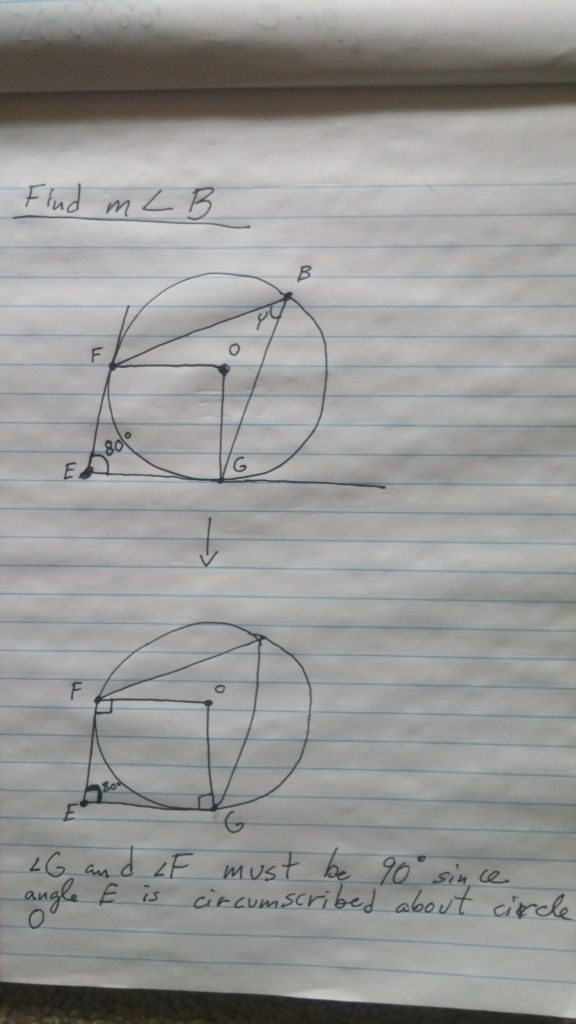# Tangents of circles

In the following question we are asked to find angle Y in the pictured circle. We are given angle E as 80° and told that the rays extending from point E are circumscribed to circle O (they are tangents to the circle).In order to find angle B we first must recall that angles F and G will be 90° because any radius segment will meet perpendicular to a tangent line. And if you recall that a quadrilateral has 360°, we know that angle O will be 100°. Since angle O is a central angle, it is also the same as the arc is forms, from F to G. Its inscribed angle B (aka Y) will be half of the central angle, thus the answer is 50°.